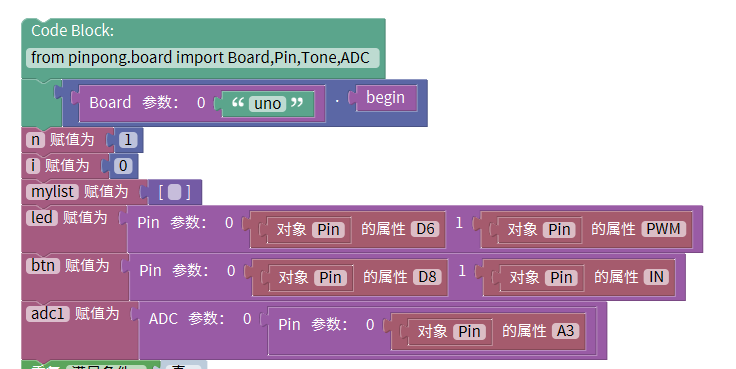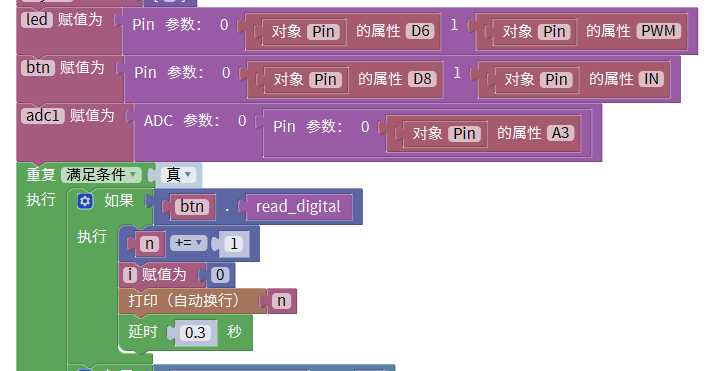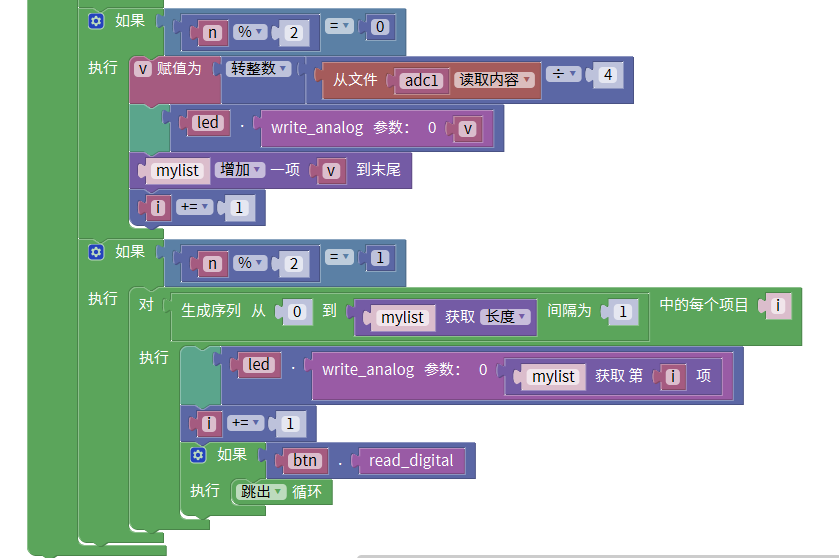[[d.project_title]][[d.material_name]]【PinPong Board】mixpy图形化项目一：会学习的智能灯（输入输出模块深入探究）

1x
pinpong board
1x
led灯
3ximport time
import math
(Board("uno")).begin()
n = 1
i = 0
mylist = []
led = Pin(Pin.D6, Pin.PWM)
btn = Pin(Pin.D8, Pin.IN)
while True:
n += 1
i = 0
print(n)
time.sleep(0.3)
if n % 2 == 0:
led.write_analog(v)
mylist.append(v)
i += 1
if n % 2 == 1:
for i in range(0, len(mylist), 1):
led.write_analog(mylist[i])
i += 1
break

Makelog作者原创文章，未经授权禁止转载。
0
0[[c.user_name]] [[c.create_time]]
[[c.parent_comment.count]]
|
[[c.comment_content]]
[[r.user.user_name]] [[r.create_time]]
[[r.comment_content]]

1
1
9
18
25
40
3
22
0
4